• 手动实现西瓜书课后习题3.3对数几率回归分类 西瓜书课后习题3.3对西瓜数据集实现对数几率回归，完成对数据集的分类，输出分类精度 import matplotlib.pyplot as plt import numpy as np import pandas as pd path =...
手动实现西瓜书课后习题3.3对数几率回归分类
西瓜书课后习题3.3对西瓜数据集实现对数几率回归，完成对数据集的分类，输出分类精度
import matplotlib.pyplot as plt
import numpy as np
import pandas as pd

path = r'C:\Users\Documents\WeChat Files\wxid_d76e8odqe93l22\FileStorage\File\2020-03\课后习题3.3_西瓜数据集3.0α.xlsx'
melon['好瓜'] = melon['好瓜'].map({'是':1,'否':0})          #将类别标签置换成0，1分类
label=np.array(melon['好瓜'])
labels=label.reshape(17,1)                                 #对标签reshape方便后续运算
data=np.array(melon[['密度','含糖率']])

def sigmoid(z):
return 1.0 / (1 + np.exp(-z))                          #定义sigmoid函数

b=np.ones((len(melon),1))
data = np.c_[b,data]									   #添加常数项

w=np.ones((3,1))                                           #初始化参数
n=0.01                                                     #学习率
for i in range(500000):
y = sigmoid(data.dot(w))
m = y-labels										   #计算误差值
w = w-data.transpose().dot(m)*n                        #误差反传更新参数，梯度下降算法
print(np.abs(m).sum())                                     #打印误差值

plt.scatter(data[:, 1], data[:, 2], c=label, cmap=plt.cm.Spectral)
x=np.linspace(0,1,100)
y=-(w*x+w)/w
plt.plot(x,y)
plt.rcParams['font.sans-serif']=['SimHei']
plt.xlabel('密度')
plt.ylabel('含糖率')
plt.show()                              #可视化，查看分类结果


误差值之和：5.903888797109145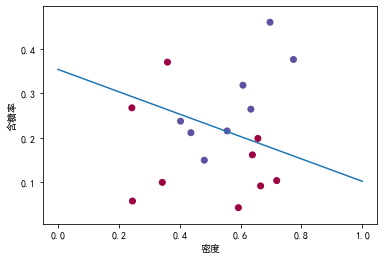可视化分类结果，直线上方为1类，下方为2类
分类准确率为70.6%
对分类结果不太满意，继续添加参数，添加密度平方项，再进行对数几率回归
import matplotlib.pyplot as plt
import numpy as np
import pandas as pd

path = r'C:\Users\Documents\WeChat Files\wxid_d76e8odqe93l22\FileStorage\File\2020-03\课后习题3.3_西瓜数据集3.0α.xlsx'
melon['好瓜'] = melon['好瓜'].map({'是':1,'否':0})
label=np.array(melon['好瓜'])
labels=label.reshape(17,1)
data=np.array(melon[['密度','含糖率']])

def sigmoid(z):
return 1.0 / (1 + np.exp(-z))

b=np.ones((len(melon),1))
data = np.c_[b,data]
x2=np.square(data[:,])
data = np.c_[data,x2]						#添加密度的平方项

w=np.ones((4,1))
n=0.01
for i in range(500000):
y = sigmoid(data.dot(w))
m = y-labels
w = w-data.transpose().dot(m)*n
print(np.abs(m).sum())

plt.scatter(data[:, 1], data[:, 2], c=label, cmap=plt.cm.Spectral)
x=np.linspace(0,1,100)
y=-(w*x+w+w*x*x)/w
plt.plot(x,y)
plt.ylim(0., 0.5)
plt.rcParams['font.sans-serif']=['SimHei']
plt.xlabel('密度')
plt.ylabel('含糖率')
plt.show()

误差值之和：4.4156484190286545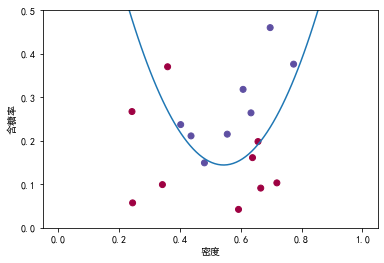分类准确率82.4%


展开全文python 机器学习 逻辑回归
• https://blog.csdn.net/kchai31/article/details/78966941 转载于:https://www.cnblogs.com/elpsycongroo/p/9823589.html
https://blog.csdn.net/kchai31/article/details/78966941
转载于:https://www.cnblogs.com/elpsycongroo/p/9823589.html
展开全文• 《机器学习》西瓜书课后习题3.3——python实现对率回归（梯度下降法） 《机器学习》西瓜书P69： 3.3 编程实现对率回归，并给出西瓜数据集3.0a上的结果 首先我们回归一下什么的是对率回归？ 对数几率回归模型，是一...
《机器学习》西瓜书课后习题3.3——python实现对率回归（梯度下降法）
《机器学习》西瓜书P69：

3.3 编程实现对率回归，并给出西瓜数据集3.0a上的结果

首先我们回归一下什么的是对率回归？
对数几率回归模型，是一个典型的二分类任务学习模型
书接上回，我们可以对一个数据集得到它的回归方程
我们是这样去解读回归方程的：在相关属性下对样例的某一特征的度量
我们根据回归方程得到的特征度量是一个连续值，我们可以根据这个度量值进行分类
例如：大学的绩点计算，当我们的绩点大于等于2.0才能拿到学位，否则拿不到学位，我们可以认为当度量值达到多少时将样例视为一类，而没达到的样例分为另一类。
但是问题就在于：

训练数据集中需要预测的特征是分类的标签（0， 1），而不是其度量值
若训练集给出了需要预测的特征的分类标签（0， 1）和度量值，但是我们无法知道两个分类的度量值的界限

根据我们的需求，提出以下假设：
我们的线性方程为：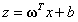设有一个函数g(x)：将我们的线性方程和这个g(x)合并可得这样我们就可利用这个函数模型来对数据分类了。使用最多的就是对数几率函数，其标准形式为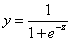我们将我们的线性回归方程与对数几率函数合并，得到我们的新模型如下：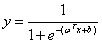我们依然使用差值来度量它的预测准确度，则我们的优化目标函数(代价函数)如下：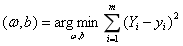我们可以使用梯度下降算法来计算上述优化目标。
梯度下降法公式如下(梯度下降法的证明推导过程见https://blog.csdn.net/qq_41398808/article/details/90442685)：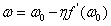我们的优化目标函数的一阶导数为（计算过程参考：https://www.cnblogs.com/crackpotisback/p/5545708.html）：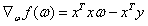所以我们根据该公式对参数进行迭代，就可以得到我们想要的结果。

【数据集】
西瓜数据集3.0a.csv：
编号,密度,含糖率,好瓜
1,0.697,0.46,是
2,0.774,0.376,是
3,0.634,0.264,是
4,0.608,0.318,是
5,0.556,0.215,是
6,0.403,0.237,是
7,0.481,0.149,是
8,0.437,0.211,是
9,0.666,0.091,否
10,0.243,0.267,否
11,0.245,0.057,否
12,0.343,0.099,否
13,0.639,0.161,否
14,0.657,0.198,否
15,0.36,0.37,否
16,0.593,0.042,否
17,0.719,0.103,否

首先我们需要读取数据并进行预处理，将属性矩阵和标记矩阵分开存储，同时在属性矩阵的最后增加全为1的一列。我在这里直接将最后一列全替换为1，并根据标记存放到label数组里面。
#读取西瓜数据集中的数据并进行预处理
dataset=[]
labelset=[]
with open(filename,'r',encoding='utf-8') as csvfile:
if row == '是':
labelset.append(1)
elif row == '否':
labelset.append(0)
row=1
dataset.append(row)
data=[[float(x) for x in row]for row in dataset]
return dataset,labelset


【对数几率函数——sigmoid】
接下来我们定义对数几率函数sigmoid
#定义sigmoid函数
def sigmoid(z):
return 1.0 / (1 + np.exp(-z))

【迭代求w函数——training】
下面我们需要采用迭代的方式求w，根据上面的公式我们进行合并化简得到最终的梯度下降公式：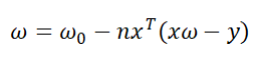其中n为步长，最终我们得到的w是一个数组，每一个元素表示的是该属性前面的系数w，为了控制迭代次数，我们将”准确率高于90%“作为终止的条件；
#迭代求w
def training(dataset,labelset):
# np.dot(a,b) a和b矩阵点乘
# np.transpose()  转置
# np.ones((m,n))  创建一个m行n列的多维数组
data=np.mat(dataset).astype(float)
label=np.mat(labelset).transpose()
w = np.ones((len(dataset),1))

#步长
n=0.0001

# 每次迭代计算一次正确率（在测试集上的正确率）
# 达到0.90的正确率，停止迭代
rightrate=0.0
while rightrate<0.90:
c=sigmoid(np.dot(data,w))
b=c-label
change = np.dot(np.transpose(data),b)
w=w-change*n
#预测，更新准确率
rightrate = test(dataset,labelset,w)

return w

【计算准确率函数——test】
因此为了计算准确率我们定义了一个函数test，作用是将每次迭代取得的w作为参数代入公式和并判断y是否大于或小于0.5，得到预测值；并与实际标记值是否相等，若相等则判断正确的个数+1，最终返回准确率。
def test(dataset,labelset,w):
data=np.mat(dataset).astype(float)

y=sigmoid(np.dot(data,w))
b,c=np.shape(y)#功能是查看矩阵或者数组的维数。
rightcount=0

for i in range(b):
flag=-1
if y[i,0]>0.5:
flag=1
elif y[i,0]<0.5:
flag=0
if labelset[i] == flag:
rightcount+=1

rightrate=rightcount/len(dataset)
return rightrate

【完整源代码】
#读取本地数据
import csv
import numpy as np

#读取西瓜数据集中的数据并进行预处理
dataset=[]
labelset=[]
with open(filename,'r',encoding='utf-8') as csvfile:
if row == '是':
labelset.append(1)
elif row == '否':
labelset.append(0)
row=1
dataset.append(row)
data=[[float(x) for x in row]for row in dataset]
return dataset,labelset

#定义sigmoid函数
def sigmoid(z):
return 1.0 / (1 + np.exp(-z))

def test(dataset,labelset,w):
data=np.mat(dataset).astype(float)

y=sigmoid(np.dot(data,w))
b,c=np.shape(y)#功能是查看矩阵或者数组的维数。
rightcount=0

for i in range(b):
flag=-1
if y[i,0]>0.5:
flag=1
elif y[i,0]<0.5:
flag=0
if labelset[i] == flag:
rightcount+=1

rightrate=rightcount/len(dataset)
return rightrate

#迭代求w
def training(dataset,labelset):
# np.dot(a,b) a和b矩阵点乘
# np.transpose()  转置
# np.ones((m,n))  创建一个m行n列的多维数组
data=np.mat(dataset).astype(float)
label=np.mat(labelset).transpose()
w = np.ones((len(dataset),1))

#步长
n=0.0001

# 每次迭代计算一次正确率（在测试集上的正确率）
# 达到0.90的正确率，停止迭代
rightrate=0.0
while rightrate<0.90:
c=sigmoid(np.dot(data,w))
b=c-label
change = np.dot(np.transpose(data),b)
w=w-change*n
#预测，更新准确率
rightrate = test(dataset,labelset,w)

return w

dataset=[]
labelset=[]
filename = '西瓜数据集3.0a.csv'
w=training(dataset,labelset)
print("若使得准确率大于90%，则此时的w为：\n",w)
print("正确率：%f"%(test(dataset,labelset,w)*100)+"%")


【运算结果】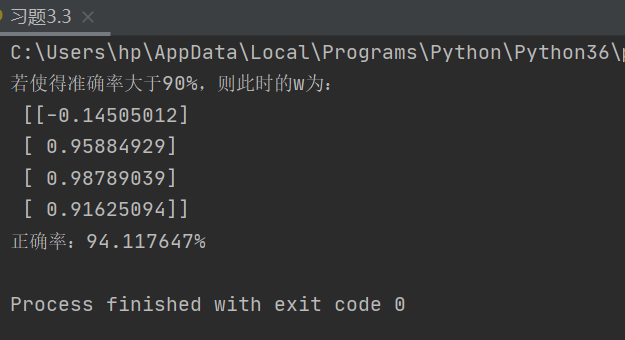所以最终得到的西瓜模型为：
$y=-0.14505012*编号+0.95884929*密度+0.98789039*含糖率+0.91625094$
参考博文：
机器学习 对数几率回归模型(Python实现)
上述解题过程如有错误 还请及时留言联系


展开全文python 机器学习
• 《机器学习》西瓜书课后习题3.5——python实现线性判别分析 《机器学习》西瓜书P69 3.5 编程实现线性判别分析，并给出西瓜数据集3.0a上的结果 理论学习参见文章：线性判别分析LDA原理总结 注意：在该文章中针对w的...
《机器学习》西瓜书课后习题3.5——python实现线性判别分析
《机器学习》西瓜书P69

3.5 编程实现线性判别分析，并给出西瓜数据集3.0a上的结果

理论学习参见文章：线性判别分析LDA原理总结
注意：在该文章中针对w的求法出现了两种方式，一种是
$w=S^-1 w(μ_0−μ_1)$
该方法指的应该是针对二类LDA，所以我们在解决西瓜数据集问题是求w的方法采用此方法。
另一种方法是：
$计算S^−1_wS_b的最大的d个特征值和对应的d个特征向量(w_1,w_2,...w_d),得到投影矩阵W$
此方法针对的解决多分类问题是采用的策略，在本问题中并不适用，具体详见下文。

二类线性判别分析的解决步骤：

求出均值向量、协方差矩阵
求散度矩阵
求出w

下面我们按照上述思路逐步完成，并绘出图像。
一. 数据的预处理
将西瓜集中的数据进行分割、修改
#读取西瓜数据集中的数据并进行预处理
dataset=[]
with open(filename,'r',encoding='utf-8') as csvfile:
if row == '是':
row=1
else:
row=0
del(row)
dataset.append(copy.deepcopy(row))
data=[[float(x) for x in row]for row in dataset]
print(data)
return data

二. LDA算法实现
关于求均值向量、协方差矩阵可参见python中numpy函数中的部分用法，在这不再赘述，直接适用。
def LDA(data):
data0=[]
data1=[]
for x in data:
if x==1:
del(x)
data1.append(copy.deepcopy(x))
else:
del (x)
data0.append(copy.deepcopy(x))

# 求得两类数据的均值向量
mean0 = np.mean(data0)
mean1 = np.mean(data1)
# 得到两种数据的协方差矩阵
diff1 = data1 - mean1
diff0 = data0 - mean0
cov1 = np.dot(np.transpose(diff1), diff1)
cov0 = np.dot(np.transpose(diff0), diff0)
# 计算类内散度矩阵
Sw = cov1 + cov0
# 计算类间散度矩阵
Sb=np.dot(np.transpose(mean0-mean1),(mean0-mean1))
Sw_Inv=np.linalg.inv(Sw)
# a,b=np.linalg.eig(np.dot(Sw_Inv,Sb))# 计算矩阵的特征值和所对应的特征向量
# index = np.argsort(-a)# 将-a中的元素从小到大排列，提取其对应的index(索引)
# maxIndex = index[:1]
# w = b[:, maxIndex]
# print(w)
w=np.dot(Sw_Inv,mean0-mean1)
print(w)
return w

注意：上面有一部分代码加以注释，在此予以说明，
a,b=np.linalg.eig(np.dot(Sw_Inv,Sb))# 计算矩阵的特征值和所对应的特征向量
index = np.argsort(-a)# 将-a中的元素从小到大排列，提取其对应的index(索引)
maxIndex = index[:1]
w = b[:, maxIndex]

这块代码是为了求在多类LDA方法中要求的特征向量和特征值，为了验证，我们也用此方法求得了一个w，也参与了后序的运算，最终得到了图像如下：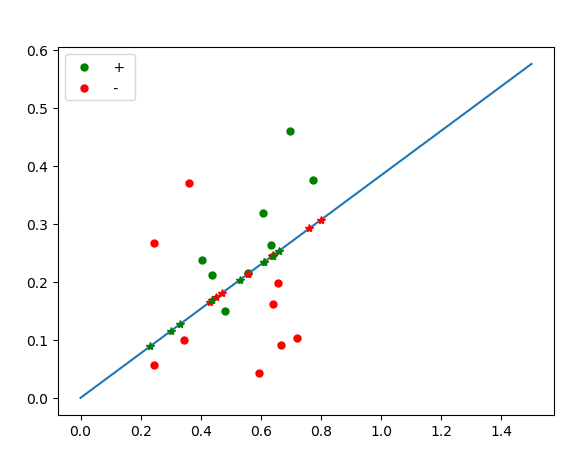很明显，所有的点并未完全得到分离，尤其是在直线上存在点的重合现象，因此使用特征向量和特征值所求得的w并不能很好的适用于二分类问题，因此抛弃该方法！
三. 绘图
def DrawGraph(dataset,w):
plt.rcParams['font.sans-serif'] = ['SimHei']
plt.rcParams['axes.unicode_minus'] = False
# matplotlib画图中中文显示会有问题，需要这两行设置默认字体

plt.xlabel('密度')
plt.ylabel('含糖量')
plt.xlim(xmax=0.8, xmin=-0.3)
plt.ylim(ymax=0.5, ymin=-0.3)
# 画两条坐标轴并设置轴标签x，y
x1=[]
y1=[]
x2=[]
y2=[]

for x in dataset:
if x==1:
x1.append(copy.deepcopy(x))
y1.append(copy.deepcopy(x))
else:
x2.append(copy.deepcopy(x))
y2.append(copy.deepcopy(x))

colors1 = '#00CED1'  # 点的颜色
colors2 = '#DC143C'
area = np.pi * 4 ** 2  # 点面积
# 画散点图
plt.scatter(x1, y1, s=area, c=colors1, alpha=0.4, label='好瓜')
plt.scatter(x2, y2, s=area, c=colors2, alpha=0.4, label='非好瓜')
plt.plot([0, 9.5], [9.5, 0], linewidth='0.5', color='#000000')

# plot line
w = w.flatten()
x1 = np.linspace(-1, 1, 102)
x2 = -w * x1 / w
plt.plot(x1, x2, label="LDA")
plt.legend()
#plt.savefig(r'C:\Users\hp\Desktop\《机器学习》笔记\LDA.png', dpi=300)
plt.show()

最终得到的图像如下：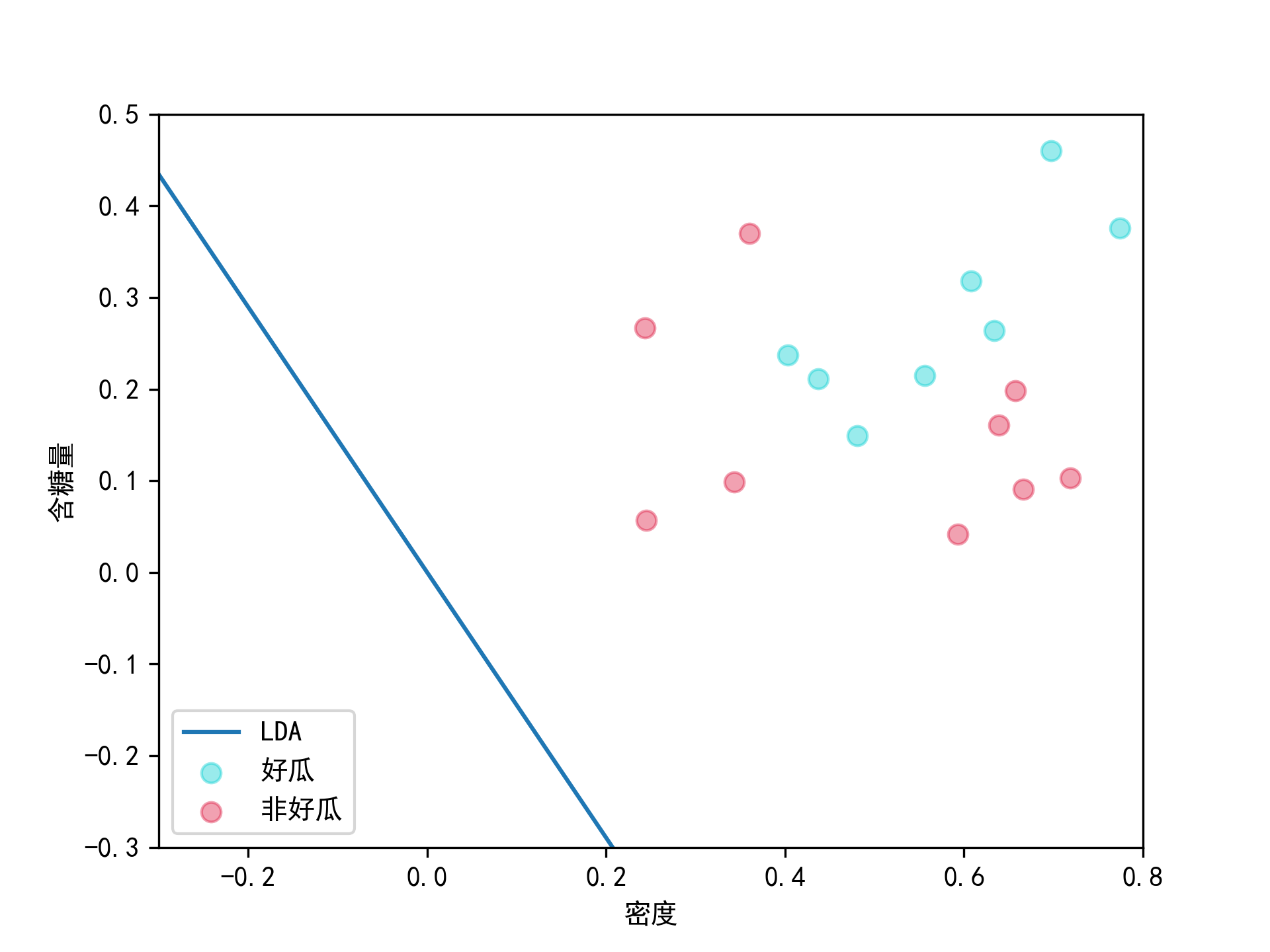展开全文• ## 周志华西瓜书课后习题答案总目录

万次阅读 多人点赞 2019-04-17 22:33:51
机器学习(周志华西瓜书)参考答案总目录 从刚开始学习机器学习到现在也有几个月了，期间看过PDF，上过MOOC，总感觉知道一点了又不是特别明白，最后趁某东买书大减价弄了几本相关的书来看看，其中一本...每章的习题都...
• 6.1 6.2 from libsvm.svm import * from libsvm.svmutil import * y = [1,1,1,1,1,1,-1,-1,-1,-1,-1,-1] ...x = [{1:0.774,2:0.376},{1:0.634,2:0.264},{1:0.608,2:0.318},{1:0.556,2:0.215},{1:0.403,2:0....
• /home/zwt/PycharmProjects/test/Machine_Learning/课后题/data_5.5.csv ' , delimiter= " , " ) attributeMap = {} attributeMap[ ' 浅白 ' ]= 0 attributeMap[ ' 青绿 ' ]=0.5 attributeMap[ ' 乌黑 ' ]=1 ...
• 3.1 式3.2 $f(x)=\omega ^{T}x+b$ 中，$\omega ^{T}$ 和b有各自的意义，简单来说，$\omega ^{T}$ 决定学习得到模型(直线、平面)的方向，而b则决定截距，当学习得到的模型恰好经过原点时，可以不考虑偏置项b。...
• 2.1 数据集包含500正例和500反例，按照70%的比例划分训练集和验证集，则验证集需要有150个正例、150个反例， 则共有$\left ( C_{500}^{150} \right )^{2}$ 种划分方式。 2.2 数据集包含100个样本，10折交叉验证...
• /home/zwt/PycharmProjects/test/Machine_Learning/课后题/tree4.4.1.png ' ) rpart.plot(dtree,branch =1,type=4,fallen.leaves=T,cex=0.8,sub= ' 剪枝前 ' ) png(file = " /home/zwt/PycharmProjects/test/...
• 1.1 若表中只包含编号1和4两个样例，则训练数据集为 色泽 根蒂 敲声 是否好瓜 青绿 蜷缩 浊响 是 乌黑 稍蜷 沉闷 否 上表有三个属性，每个属性有两种取值，所以上述......
• import csv import numpy as np import matplotlib.pyplot as plt def newton(X, y): """ Input: X: np.array with shape [N, 3]. Input. y: np.array with shape [N, 1].... Re...机器学习 对率回归
• 机器学习（周志华）西瓜书 课后习题4.3 信息熵决策树算法——python实现 算法原理 1.信息熵 2.信息增益 我们所以要做的就是不断地从当前剩余的属性当中选取最佳属性对样本集进行划分。 算法的伪代码 数据集 ...机器学习 python 决策树 算法
• 《机器学习》西瓜书课后习题3.4——python解交叉验证和留一法的对率回归错误率 《机器学习》西瓜书P69 3.3 选择两个UCI数据集，比较10折交叉验证法和留一法所估计出的对率回归的错误率 数据集：鸢尾花数据集 数据...机器学习
• 《机器学习》西瓜书课后习题4.3——python实现基于信息熵划分的决策树算法 《机器学习》西瓜书P93 4.3 试编程实现基于信息熵进行划分选择的决策树算法，并为表4.3中数据生成一棵决策树。 以下内容是使用sklearn...
• import numpy as np def dataSet(): ''' 西瓜3.0数据集离散化 ''' X = np.mat('2,3,3,2,1,2,3,3,3,2,1,1,2,1,3,1,2;\ 1,1,1,1,1,2,2,2,2,3,3,1,2,2,2,1,1;\ 2,3,2,3,2,2,2,2,3,1...机器学习 bp算法
• 习题1.1 假设空间：所有假设组成的空间，学习的过程可以看作一个在假设空间中进行搜索的过程，搜索的目标是找到与训练集匹配的假设。 版本空间：一个与训练集一致的“假设集合”。也就是说针对某个有限的训练集，......